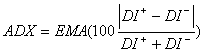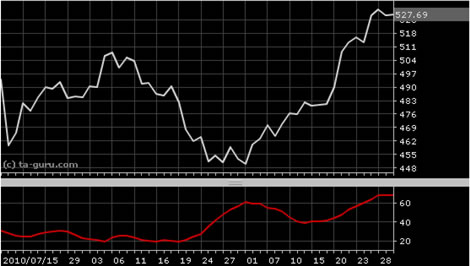Chart Pro online
User name (email):

 Recently used

 Forex

 Indices

# Average Directional Index (ADX)

Average Directional Index (ADX) was created by J. Welles Wilder as indicator of trend strength. It is important to identify if market is trending or it is moving sideways (no trend). That's why ADX become widely used indicator in technical analysis.

ADX is calculated by formula:Average directional index is calculated using directional indicators: (positive directional indicator (DI+) and negative directional indicator (DI-)) and formula of exponential moving average (EMA). For ADX period is usually used value of 14.Average Directional Index (ADX)

Interpretation

ADX measures strength of trend. Its value is limited between 0 and 100. Value higher than 40 indicates that trend is strong, and value below 20 indicates that there is no trend (price is moving sideways).

Example